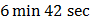# A steady current is set up in a metallic wire of non-uniform cross-section. How is the rate of flow of electrons () related to the area of cross-section ()? a)b)c)d)is independent of## Question ID - 150378 :- A steady current is set up in a metallic wire of non-uniform cross-section. How is the rate of flow of electrons () related to the area of cross-section ()? a)b)c)d)is independent of3537

 If 96500of electricity liberates one gram equivalent of any substance, the time taken for a current ofto depositof copper from a solution of copper sulphate is (Chemical equivalent of copper = 32) a)b)c)d)